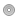Fossegrim0Just a Number 17 8, 1:45pm

The Danish system is only a little weird once you get to 50. Before 50 it's just like the German counting system. After 50, the numbers are said like an equation only abbreviated keeping in mind there is always a multiplication of 20. for example 56 is seksoghalvtreds which = 6+((3 - 0.5)x 20)=56. Halvtreds in this case is implying 3 minus 1/2 of one (or 0.5). Another example 87. Syvogfirs = 7+(4X20) =87. Notice there is no halvs because 80 is evenly divisible by 20. Nioghalvfems is 99. 9+((5 - 0.5) x 20) = 99 And so on and so on.